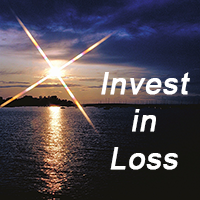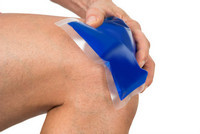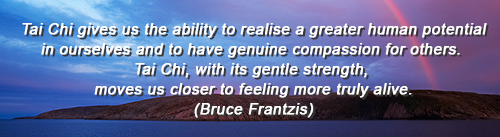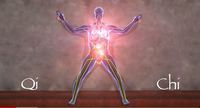Like   Tweet   Pin   +1   in
 /* styles */ Tai Chi Stories, Tips and Resources   ||   Issue #22 Decenber 2019
 table div table+table+table div table{width:100%;padding:0}table div table+table+table div table img{width:96.23%;padding:0;float:none}table div table+table+table div table td{width:100%;padding:0 1.88% 18px}/* styles */Welcome to our last issue for what's been a very busy year!

I've shared a favourite quote I find helpful in my own Tai Chi practice and for success in any sport or contest.

There are two video clips, one about the benefits of Tai Chi for those with chronic heart failure, and a second explaining the physiology of Tai Chi and Qigong.

And, as well as the usual insipriation quote, I've also included a tip to protect your knees when doing Tai Chi.

Until the next issue, I'll also wish you all the best for a festive holiday season and a great start to the new year! :)

Regards
Chris Bennett
Chris Chi

 table div table+table+table+table+table div table{width:100%;padding:0}table div table+table+table+table+table div table img{width:96.23%;padding:0;float:none}table div table+table+table+table+table div table td{width:100%;padding:0 1.88% 18px}/* styles */## Sports Chi - 'Invest In Loss'In my Sports Chi program, one of the areas we cover is the practical meaning of various Chinese proverbs and/or quotes that can help athletes improve their performance.

One of my favourite quotes is 'invest in loss', which is thought provoking because it makes you think on a different level when it comes to success in contests and games.

 table div table+table+table+table+table+table+table+table div table{width:100%;padding:0}table div table+table+table+table+table+table+table+table div table img{width:96.23%;padding:0;float:none}table div table+table+table+table+table+table+table+table div table td{width:100%;padding:0 1.88% 18px}/* styles */## Tai Chi in Patients With Chronic Heart Failure

 table div table+table+table+table+table+table+table+table+table+table div table,table.module-9{width:37.74%;float:right;padding:0}table div table+table+table+table+table+table+table+table+table+table div table a{border:0 none;text-decoration:none}table div table+table+table+table+table+table+table+table+table+table div table img{width:100%!important;border:0 none;text-decoration:none}table div table+table+table+table+table+table+table+table+table+table div table td{width:100%;padding:0 0 20px 20px}/* styles */ In this clip Dr Gloria Yeh briefly covers the benefits that Tai Chi may have for those who have a 'perceived dyspnea, one of the main symptoms in heart failure'. She says that Tai Chi includes aerobic exercise, core strength, upper and lower body, a mind and body component, and an emphasis on breathing control.
 table div table+table+table+table+table+table+table+table+table+table+table div table{width:100%;padding:0}table div table+table+table+table+table+table+table+table+table+table+table div table img{width:96.23%;padding:0;float:none}table div table+table+table+table+table+table+table+table+table+table+table div table td{width:100%;padding:0 1.88% 18px}/* styles */## Tai Chi TipTo help avoid knee strain, keep your kness pointed towards your toes when moving or standing still.

Make sure the knees don't go over the toes.

 table div table+table+table+table+table+table+table+table+table+table+table+table+table+table div table{width:100%;padding:0}table div table+table+table+table+table+table+table+table+table+table+table+table+table+table div table img{width:96.23%;padding:0;float:none}table div table+table+table+table+table+table+table+table+table+table+table+table+table+table div table td{width:100%;padding:0 1.88% 18px}/* styles */table div table+table+table+table+table+table+table+table+table+table+table+table+table+table+table+table div table{width:100%;padding:0}table div table+table+table+table+table+table+table+table+table+table+table+table+table+table+table+table div table img{width:96.23%;padding:0;float:none}table div table+table+table+table+table+table+table+table+table+table+table+table+table+table+table+table div table td{width:100%;padding:0 1.88% 18px}/* styles */This week I'm running a special one hour presentation for fifty grade five students at a primary school.

It's part of the Pozitive Kidz are happy kidz program I run, and in this session the emphasis will be on leadership, self esteem and confidence.

All the skills I teach are based on Tai Chi. I simply adapt them to suit the various age groups I work with - from 5 years old to 103 years old!

 table div table+table+table+table+table+table+table+table+table+table+table+table+table+table+table+table+table+table+table div table{width:100%;padding:0}table div table+table+table+table+table+table+table+table+table+table+table+table+table+table+table+table+table+table+table div table img{width:96.23%;padding:0;float:none}table div table+table+table+table+table+table+table+table+table+table+table+table+table+table+table+table+table+table+table div table td{width:100%;padding:0 1.88% 18px}/* styles */## The Physiology of Tai Chi and QiGongThis clip by Dr Roger Jahnke, OMD is a good overview of the role Chi plays in good health.

It's 12.04 minutes long, and you may find the background music a little distracting for an informational video.

But it's still very worthwhile watching to get a Chinese perspective of Chi!

 table div table+table+table+table+table+table+table+table+table+table+table+table+table+table+table+table+table+table+table+table+table+table div table{width:100%;padding:0}table div table+table+table+table+table+table+table+table+table+table+table+table+table+table+table+table+table+table+table+table+table+table div table img{width:96.23%;padding:0;float:none}table div table+table+table+table+table+table+table+table+table+table+table+table+table+table+table+table+table+table+table+table+table+table div table td{width:100%;padding:0 1.88% 18px}/* styles *//* styles */
 table div table+table+table+table+table+table+table+table+table+table+table+table+table+table+table+table+table+table+table+table+table+table+table+table div table{width:100%;padding:0}table div table+table+table+table+table+table+table+table+table+table+table+table+table+table+table+table+table+table+table+table+table+table+table+table div table img{width:96.23%;padding:0;float:none}table div table+table+table+table+table+table+table+table+table+table+table+table+table+table+table+table+table+table+table+table+table+table+table+table div table td{width:100%;padding:0 1.88% 18px}/* styles */